# Solution sets of linear systems#### Everything You Need in One Place

Homework problems? Exam preparation? Trying to grasp a concept or just brushing up the basics? Our extensive help & practice library have got you covered.#### Learn and Practice With Ease

Our proven video lessons ease you through problems quickly, and you get tonnes of friendly practice on questions that trip students up on tests and finals.#### Instant and Unlimited Help

Our personalized learning platform enables you to instantly find the exact walkthrough to your specific type of question. Activate unlimited help now!##### Intros
###### Lessons
1. Solution Set of Linear Systems Overview:
2. Homogeneous Systems
$Ax=0$
• Trivial, non-Trivial, and general solutions
3. Solution Sets of Homogeneous Systems
• Parametric Vector Equation with 1 free variable: $x=tv$
• Parametric Vector Equation with 2 free variable $x=su+tv$
• Parametric Vector Equation with more than 2 free variables
4. NonHomogeneous Systems
$Ax=b$
• General Solutions with an extra vector
5. Solution Sets of Nonhomogeneous Systems
• Parametric Vector Equation with 1 free variable: : $x=p+tv$
• Parametric Vector Equation with 2 free variables: $x=p+su+tv$
• Parametric Vector Equation with more than 2 free variables
6. Difference Between Homogeneous and Nonhomogeneous
• Extra $p=$ Translation
##### Examples
###### Lessons
1. Solution Sets of Homogeneous Systems
Find the solution set of the homogeneous system in parametric vector form:
$x_1+3x_2+3x_3=0$
$-x_1-3x_2-3x_3=0$
$2x_2+2x_3=0$
1. Find the solution set $Ax=0$ in parametric vector form if: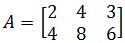1. Solution Sets of Non-homogeneous Systems
Find the solution set of the nonhomogeneous system in parametric vector form:
$x_1+2x_2-3x_3=2$
$2x_1+x_2-3x_3=4$
$-x_1+x_2=-2$
1. Comparing Homogeneous and Nonhomogeneous Systems
Describe and compare the solution sets of $2x_1+3x_2-5x_3=0$ and $2x_1+3x_2-5x_3=4$
1. Describe and compare the solution sets of $x_1-4x_2+2x_3=0$ and $x_1-4x_2+2x_3=-3$
1. Parametric equation of a line and Translation
Find the parametric equation of the line through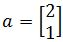parallel to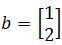. Draw all the vectors and the line to show you obtained the line itself geometrically.

## Solution Sets of linear systems

#### What is a solution set in algebra

A solution set in algebra refers to the set of values which inputted into a system of equations correctly solve such system. In other words, these values satisfy the equality of the equations contained in the system we are trying to solve and so, the list of the corresponding value for each unknown variable in a system conforms the complete set of solutions.

At this point, we are no strangers to the idea of solving a set of linear equations, we have done it in different ways through past lessons in our Linear Algebra course, such as in the lesson on solving systems of linear equations by graphing or the lesson about solving a linear system with matrices using Gaussian elimination.

We will continue to use such techniques (mostly Gaussian elimination, also known as row reduction) throughout our lesson of today to find a linear system solution, but before we continue on to that it is imperative we finally get to know the types of linear systems of equations that we can find in our problems:

A system of linear equations is homogeneous if we can write its matrix equation in the form $Ax=0$. On the other hand, a system of linear equations is nonhomogeneous if we can write its matrix equation in the form $Ax=b$.

We can express solution sets of linear systems in parametric vector form, but the type of system we have to work with will make a difference in the type of solutions we can obtain:
• Types of solutions a homogeneous system can have in parametric vector form:
• With 1 free variable: $x=tv$
• With 2 free variables: $x=su+tv$
• With n free variables: $x=av_{1}+bv_{2}+...+nvn$

• Types of solutions a nonhomogeneous system can have in a parametric vector form:
• With 1 free variable: $x=p+tv$
• With 2 free variables: $x=p+su+tv$
• With n free variables: $x=p+av_{1}+bv_{2}+...+nv_{n}$

Where x represents the column vector containing all of the corresponding unknown variables from the linear system. $u$, $v$ and $v_{1, 2 ... n}$ represent the column vectors formed by the coefficients of each unknown variable in each equation from the system; while $s$, $t$ and $a$,$b$ , ... ,$n$ are the free variables.

For the case of nonhomogeneous systems you will have an extra vector coming out in the solutions, this extra vector is what we have named $p$ above.

#### How to find a solution set of an equation

The basic steps to follow when calculating solution sets for a system of linear equations are:
1. Convert the linear system into a matrix equation
2. Rewrite the matrix equation as an augmented matrix
3. Compute the reduced echelon form of the augmented matrix using Gaussian elimination
4. The reduced echelon form of the matrix will provide the values (or expressions) for the unknown variables, use these solutions to write down the parametric vector equation form which will contain the complete set of solutions for the system.

All of these steps will be explained in detail in the exercise examples provided in the last section of this lesson. For now, it is important that if you have any doubt on how to work through any of the steps mentioned above, you revisit the next past lessons: representing a linear system as a matrix, row reduction and echelon forms and matrix equation $Ax=b$.

Before we continue onto the next section of this lesson, let us have a quick review of something we have been mentioning many times throughout our lessons: matrix multiplication!
Below you can find the diagram containing the general rules for matrix multiplication. You must be already familiarized with this diagram since we have included it in lessons before. The reason why we bring this one up, is that in order to produce matrix equations we will be looking into matrix multiplications (the matrix equations $Ax=0$ and $Ax=b$ are matrix multiplications themselves!), and so, just take the next diagram as a reference for later parts of this lesson where you will be working on exercise examples.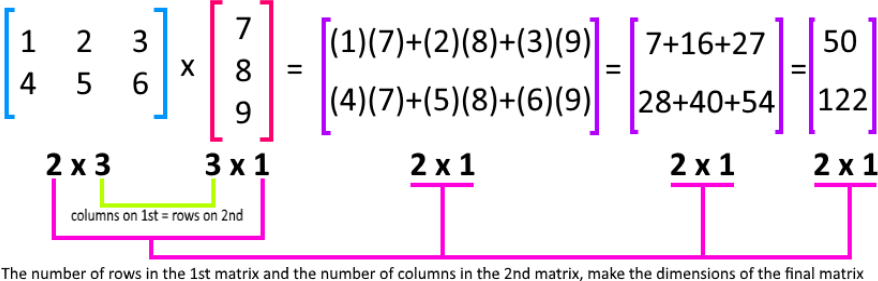#### What is a trivial solution in linear algebra

A vector is called trivial if all its coordinates are 0, for example, if it is the zero vector
In Linear Algebra we are not interested in only finding one solution to a system of linear equations. We are interested in all possible solutions. In particular, homogeneous systems of equations (see above) are very important. The important question for a homogeneous system is whether or not there is any non-trivial solution, i. e. whether there is any solution other than the trivial one. Sometimes there will be, sometimes there wont. Paradoxically, its actually the case where the trivial solution is the only possible one that is the most important. This situation is described by one of the most important words in the whole course.

#### Find solution set

For this final section of our lesson of today, we will be looking for the solutions sets of linear systems. As usual, we will be using notation of matrices in order to find the corresponding matrix solution set required in each case.

#### Example 1

Find the solution set of the homogeneous system in parametric vector form: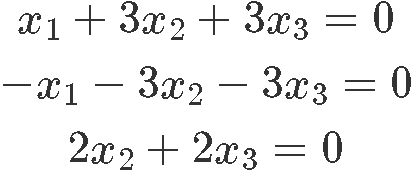As we have been doing so for a few lessons now, we transform this system into an augmented matrix and then row reduce it until we obtain its reduced echelon form in order to find the final expressions for the variables of the system: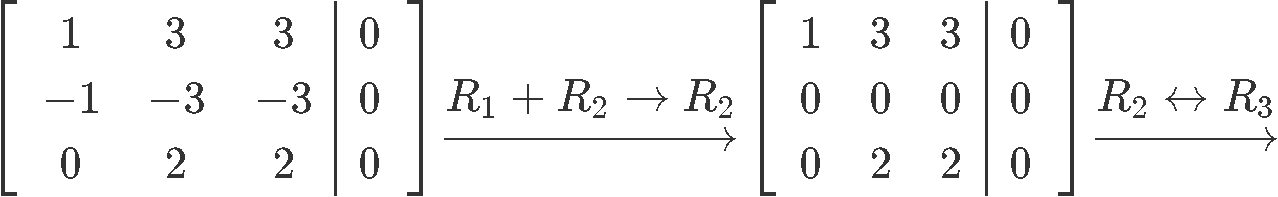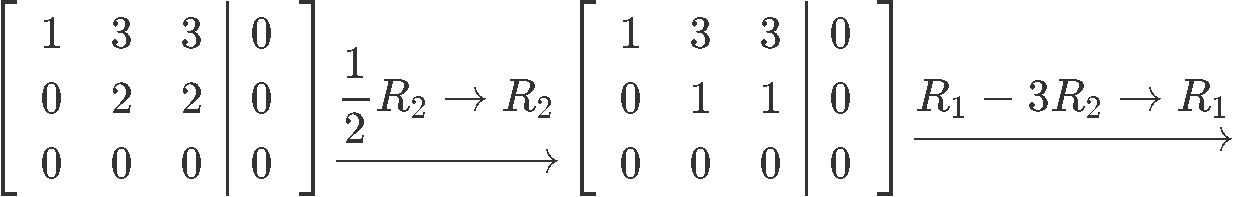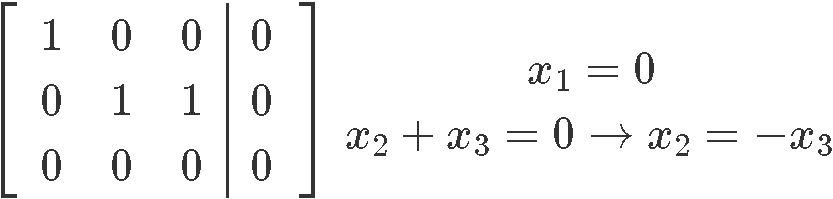As you can see from the results above, $x_{3}$ happens to be a free variable since there is no pivot corresponding to its column in the augmented matrix. And so, the final solution set for system of equations shown in equation x is: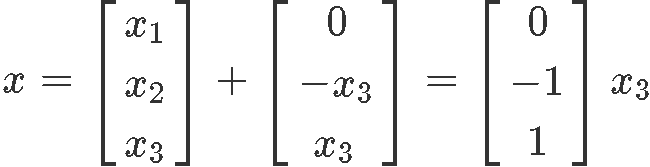#### Example 2

Given matrix $A$, find the solution set $Ax=0$ in parametric vector form: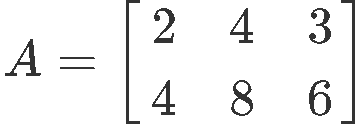We write down the matrix equation $Ax=0$ following the rules of matrix multiplication: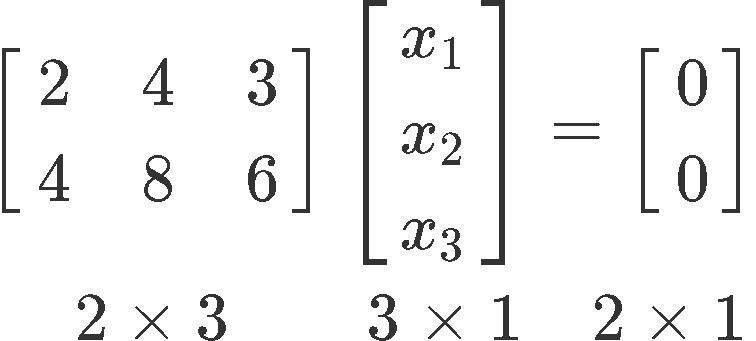We use this matrix equation to write an augmented matrix which we can solve for the unknown variables as follows: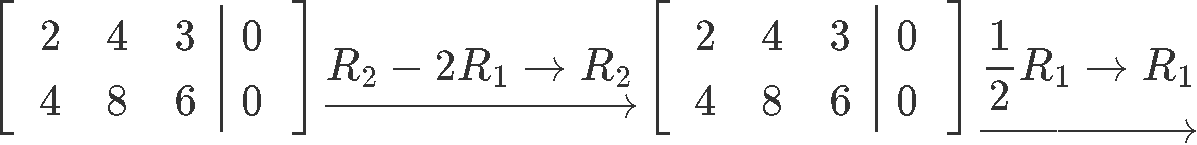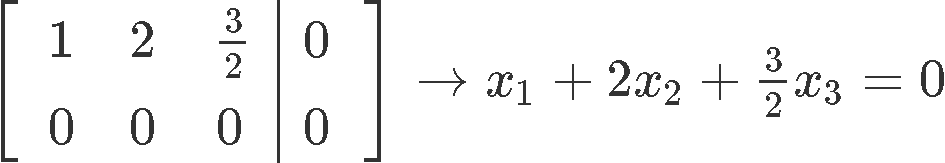By computing the reduced echelon form of the augmented matrix we can observe how we are left with no pivots for the second and third column, which means the variables $x_{2}$ and $x_{3}$ are free variables. And so, we write the solution set of linear equation 8 in parametric form as shown below: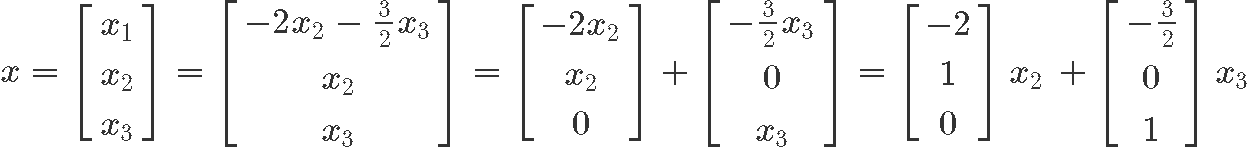#### Example 3

Find the solution set for the nonhomogeneous system in parametric vector form: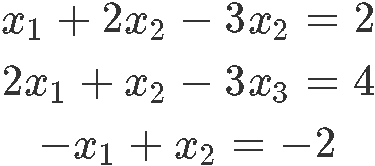Writing down the corresponding matrix equation for the linear system: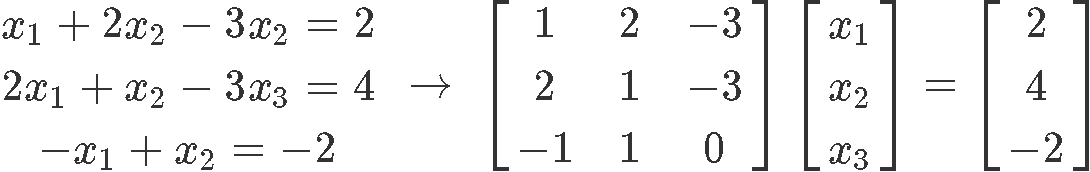We convert this matrix equation into an augmented matrix and row reduce it into we can obtain the unknown variables expressions: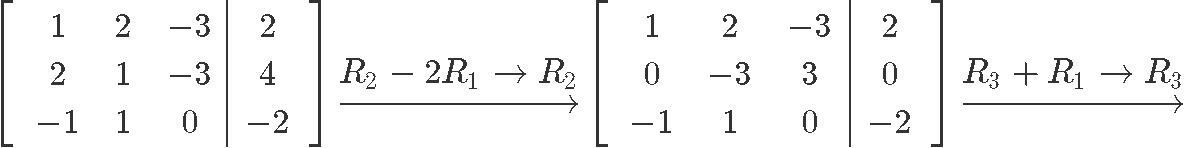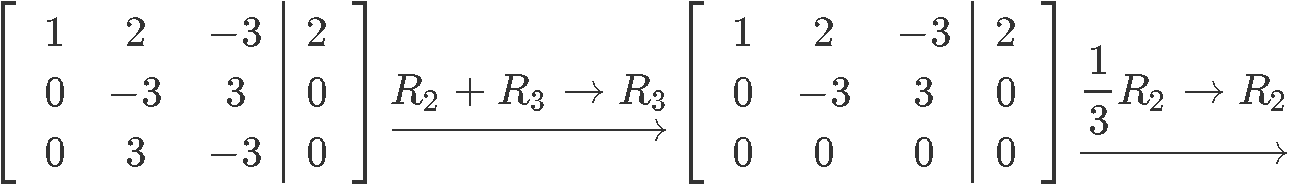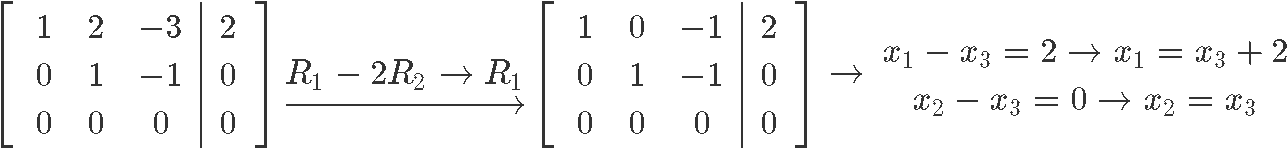As you can see, the variable $x_{3}$ is a free variable and so the final solution set for this nonhomogeneous system in parametric form is: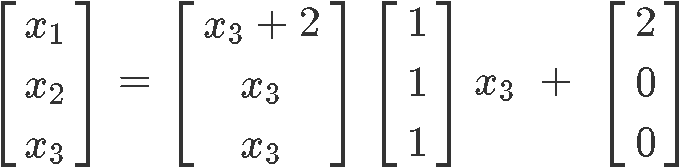Notice how our final solution set contains an extra vector without a variable factor. This is usually the case for nonhomogeneous systems as you will see in the next examples.

#### Example 4

Describe and compare the solution sets for each of the nest two linear equations:

$2x_{1} + 3x_{2} - 5x_{3} = \,$0$\;$ and $\; 2x_{1} + 3x_{2} - 5x_{3} = \,$4

For this case, each equation happens to have its own solution set, and thus, we can obtain a matrix equation, then an augmented matrix to finally arrive to the parametric vector form of the solution set with each equation.
Since we will be solving for the solution set of a linear equation at a time, let us begin with the homogeneous equation (the one on the left in equation w). We start by writing down its corresponding matrix equation: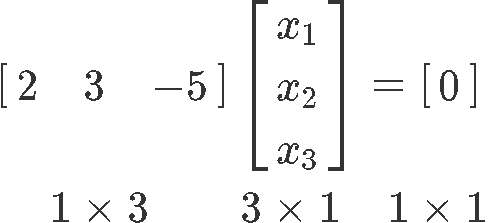Although making a matrix equation out of a single algebraic equation may seem trouble some, we just have to follow the rules of matrix multiplication shown in equation ____ to construct the equation as has been shown above. The numbers in the bottom of the equation represent the dimensions of each element in the multiplication: The first factor in the multiplication (the left-most matrix) has one row and three columns, followed by the next factor with three rows and one column. This makes sense since in order to multiply two matrices, the first one must contain the same number of columns as the second one has rows. And then, the result of such multiplication must always have the dimensions coming from the number of rows from the first matrix and the number of columns from the second matrix.

Then, the augmented matrix corresponding to the matrix equation above looks like: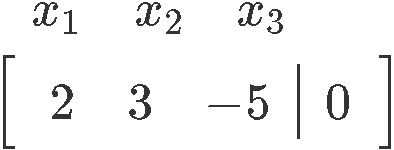Notice we have written the names of the variables being represented in each column of the matrix, this, just as the dimensions of the matrices in equation 14, is not needed. We have elected to write these specifications down so the computations can be shown clearly.
From the augmented matrix we can conclude that the variables $x_{2}$ and $x_{3}$ are free variables. And so, we write down the final solution set for this homogeneous equation: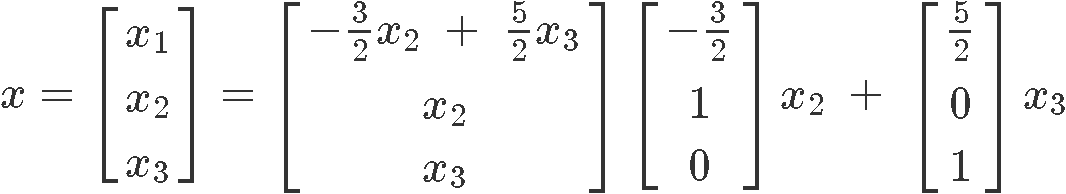Now let us obtain the linear solution of the nonhomogeneous equation (the right-most equation from the set found in equation w) in parametric vector form. We start by writing down its corresponding matrix equation: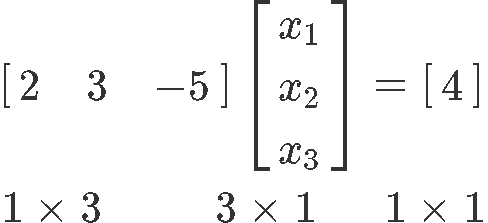Which will produce the augmented matrix below:And the solution in parametric vector form is: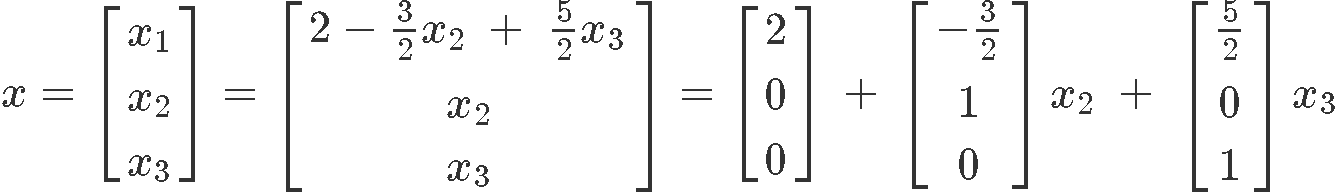Notice that the difference between the two solutions (equations 16 and 19) is only an extra vector resulting from the nonzero value of the nonhomogeneous equation.

#### Example 5

For this problem we are going to follow the exact same process as we did in the past example to solve the set of linear equations provided. To facilitate the comparison between the two results, we have designed a table in which we have inputted the computation result of each step of the process:

$x_{1} - 4x_{2} + 2x_{3} = \,$0$\;$ and $\; x_{1} - 4x_{2} + 2x_{3} = \,$-3

Now let us go through the entire process of the solution set in algebra inside the next table: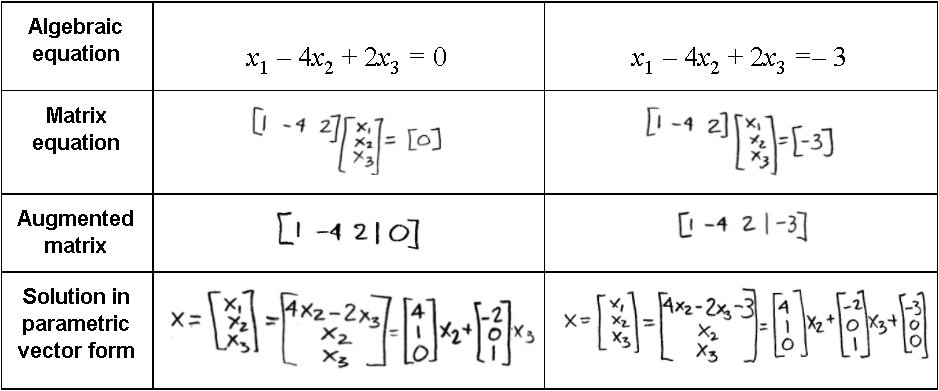Notice that variables $x_{2}$ and $x_{3}$ are free variables. And the difference between the solution of each equation is that the nonhomogeneous equation produces an extra column vector on its parametric solution.

***

To continue the practice on solving a set of linear equations we recommend you to take a look at these algebra notes on solutions and solution sets of linear systems, both links contain some more examples similar to the ones we have studied today.

It is the end of this lesson, see you in the next one!
A system of linear equations is homogeneous if we can write the matrix equation in the form $Ax=0$.

A system of linear equations is nonhomogeneous if we can write the matrix equation in the form $Ax=b$.

We can express solution sets of linear systems in parametric vector form.

Here are the types of solutions a homogeneous system can have in parametric vector form:
1. With 1 free variable: $x=tv$
2. With 2 free variables: $x=su+tv$
3. With n free variables: $x=av_1+bv_2+\cdots+nv_n$

Here are the types of solutions a nonhomogeneous system can have in a parametric vector form:
1. With 1 free variable: $x=p+tv$
2. With 2 free variables: $x=p+su+tv$
3. With n free variables: $x=p+av_1+bv_2+\cdots+nv_n$